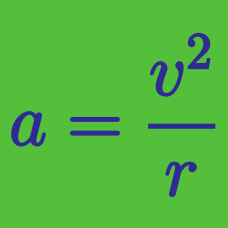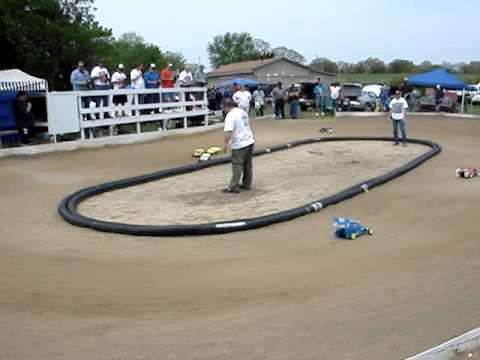Classical Mechanics

# Uniform circular motion - BasicA racing car moving at a constant tangential speed of $44 \text{ m/s}$ on a circular track takes one lap around the track in $45 \text{ seconds.}$ Determine the approximate magnitude of the acceleration of the car.

A $11 \text{ kg}$ rock swings in a circle of radius $10 \text{ m}$ at a constant speed of $7 \text{ m/s}.$ What is the centripetal acceleration?

A $5 \text{ kg}$ rock swings in a vertical circle of radius $2 \text{ m}.$ The speed of the rock as it passes its lowest point is $10 \text{ m/s}.$ What is the tension $T$ acting on the rock as it passes its lowest point?

The gravitational acceleration is $g= 10 \text{ m/s}^2.$

An object that moves in uniform circular motion has a centripetal acceleration of $11 \text{ m/s}^2.$ If the radius of the motion is $0.02 \text{ m},$ what is the approximate frequency of the motion?

A skater is skating at $14 \text{ m/s}$ on a circle of radius $4 \text{ m}.$ The ice exerts a central force of $2058 \text{ N}$ on the skater. What is the mass of the skater?

×